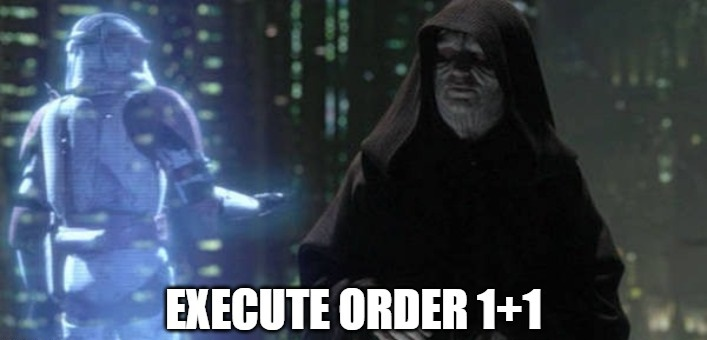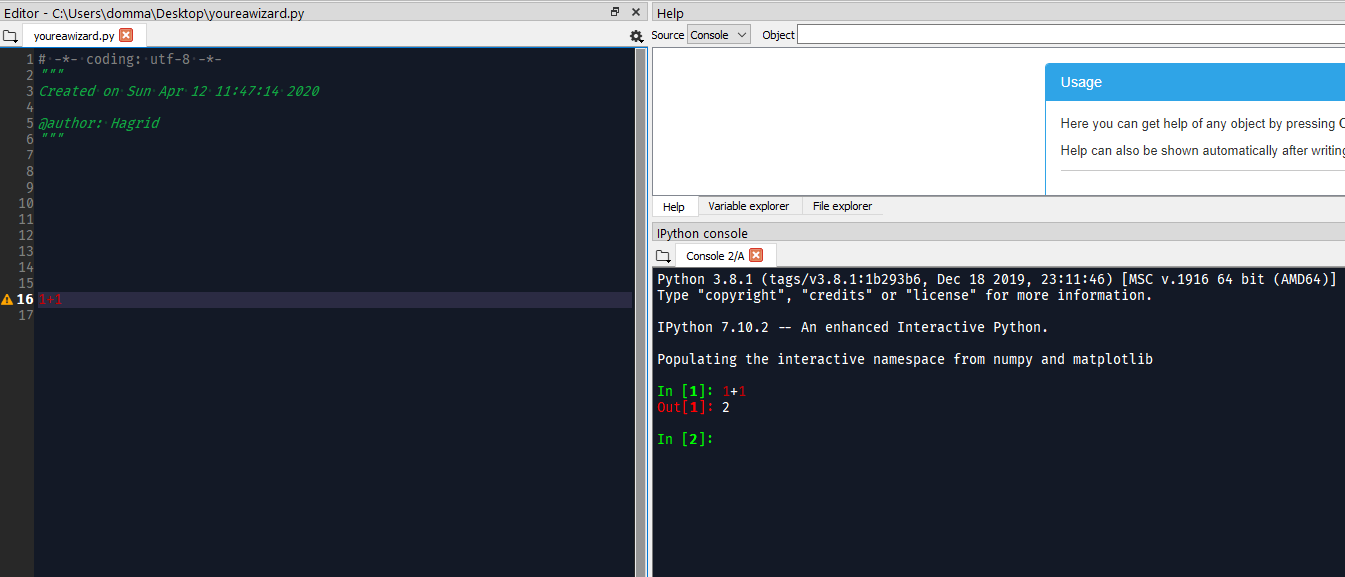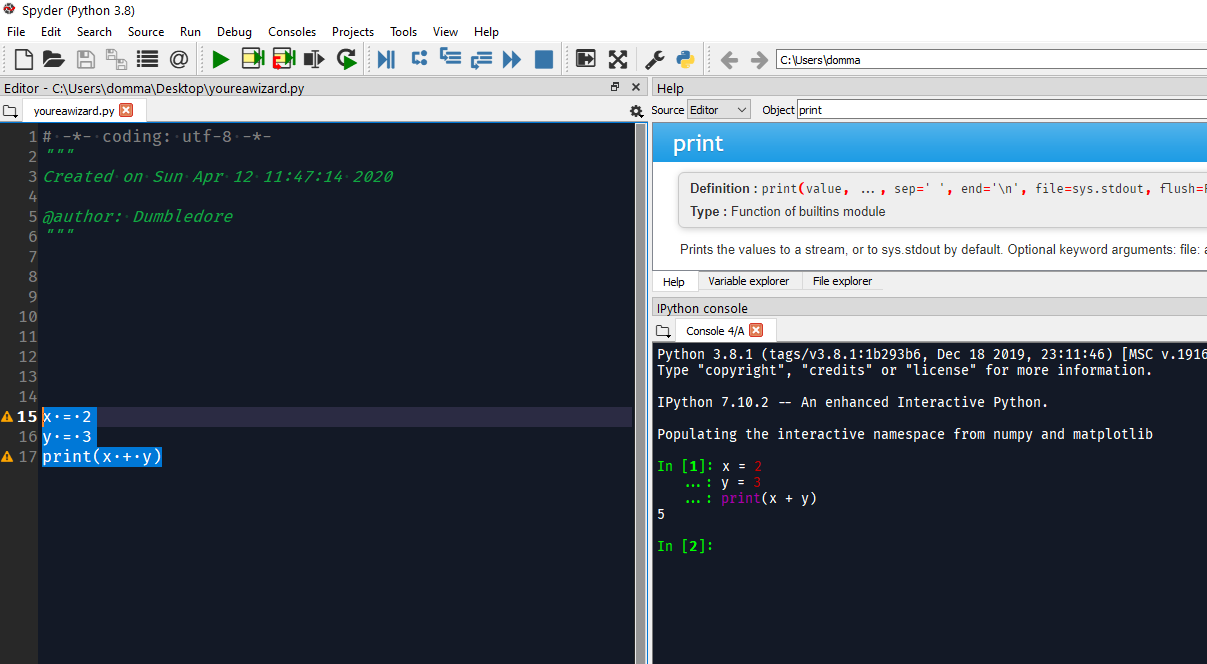# Get familiar with Python in 10 minutes¶

Hint

Spotted a typo? Would like to add something or make a correction? Join us by contributing (see these guides).

You have no experience in computer science? You are afraid of code? You feel betrayed because you didn’t expect to do programming in psychology studies? Relax! We got you covered.

This tutorial will provide you with all you need to know to dive into the wonderful world of scientific programming. The goal here is not become a programmer, or a software designer, but rather to be able to use the power of programming to get scientific results.

## Setup¶

The first thing you will need is to install Python on your computer (we have a tutorial for that). In fact, this includes two things, installing Python (the language), and an environment to be able to use it. For this tutorial, we will assume you have something that looks like Spyder (called an IDE). But you can use jupyter notebooks, or anything else, it doesn’t really matter.

There is one important concept to understand here: the difference between the CONSOLE and the EDITOR. The editor is like a cooking table where you prepare your ingredients to make a dish, whereas the console is like the oven, you only open it to put the dish in it and get the result.

Most of the code that you will write, you will write it in the editor. It’s basically a text editor (such as notepad), except that it automatically highlights the code. Importantly, you can directly execute a line of code (which is equivalent to copy it and paste it the console).

For instance, try writing `1+1` somewhere in the file in the editor pane. Now if select the piece of code you just wrote, and press `F9` (or `CTRL + ENTER`), it will execute it.As a result, you should see in the console the order that you gave and, below, its output (which is `2`).

Now that the distinction between where we write the code and where the output appears is clear, take some time to explore the settings and turn the editor background to BLACK. Why? Because it’s more comfortable for the eyes, but most importantly, because it’s cool 😎.Congrats, you’ve become a programmer, a wizard of the modern times.

You can now save the file (`CTRL + S`), which will be saved with a `.py` extension (i.e., a Python script). Try closing everything and reopening this file with the editor.

## Variables¶

The most important concept of programming is variables, which is a fancy name for something that you already know. Do you remember, from your mathematics classes, the famous x, this placeholder for any value? Well, x was a variable, i.e., the name refering to some other thing.

Hint

Despite to what I just said, a variable in programming is not equivalent to a variable in statistics, in which it refers to some specific data (for instance, age is variable and contains multiple observations). In programming, a variable is simply the name that we give to some entity, that could be anything.

We can assign a value to a variable using the `=` sign, for instance:

```x = 2
y = 3
```

Once we execute these two lines, Python will know that `x` refers to `2`, and `y` to `3`. We can now write:

```print(x + y)
```

Which will print in the console the correct result.We can also store the output in a third variable:

```x = 2
y = 3
anothervariable = x * y
print(anothervariable)
```

## Variables and data types¶

The next important thing to have in mind is that variables have types. Basic types include integers (numbers without decimals), floats (numbers with decimals), strings (character text) and booleans (`True` and `False`). Depending on their type, the variables will not behave in the same way. For example, try:

```print(1 + 2)
print("1" + "2")
```

What happened here? Well, quotations (`"I am quoted"`) are used to represent strings (i.e., text). So in the second line, the numbers that we added were not numbers, but text. And when you add strings together in Python, it concatenates them.

One can change the type of a variable with the following:

```int(1.0)  # transform the input to an integer
float(1)  # transform the input to a float
str(1)  # transform the input into text
```

Also, here I used the hashtag symbol to make comments, i.e., writing stuff that won’t be executed by Python. This is super useful to annotate each line of your code to remember what you do - and why you do it.

Types are often the source of many errors as they usually are incompatible between them. For instance, you cannot add a number (`int` or `float`) with a character string. For instance, try running `3 + "a"`, it will throw a `TypeError`.

## Lists and dictionnaries¶

Two other important types are lists and dictionnaries. You can think of them as containers, as they contain multiple variables. The main difference between them is that in a list, you access the individual elements that it contains by its order (for instance, “give me the third one”), whereas in a dictionary, you access an element by its name (also known as key), for example “give me the element named A”.

A list is created using square brackets, and a dictionary using curly brackets. Importantly, in a dictionary, you must specify a name to each element. Here’s what it looks like:

```mylist = [1, 2, 3]
mydict = {"A": 1, "B": 2, "C": 3}
```

Keep in mind that there are more types of containers, such as arrays and dataframes, that we will talk about later.

## Basic indexing¶

There’s no point in storing elements in containers if we cannot access them later on. As mentioned earlier, we can access elements from a dictionary by its key within square brackets (note that here the square brackets don’t mean list, just mean within the previous container).

```mydict = {"A": 1, "B": 2, "C": 3}
x = mydict["B"]
print(x)
```

Exercice time! If you have followed this tutorial so far, you should be able to guess what the following code will output:

```mydict = {"1": 0, "2": 42, "x": 7}
x = str(1 + 1)
y = mydict[x]
print(y)
```

Answer: If you guessed 42, you’re right, congrats! If you guessed 7, you have likely confused the variable named `x` (which represents 1+1 converted to a character), with the character `"x"`. And if you guessed 0… what is wrong with you?

## Indexing starts from 0¶

As mentioned earliers, one can access elements from a list by its order. However, and there is very important to remember (the source of many beginner errors), in Python, the order starts from 0. That means that the first element is the 0th.

So if we want the 2nd element of the list, we have to ask for the 1th:

```mylist = [1, 2, 3]
x = mylist
print(x)
```

## Control flow (if and else)¶

One important notion in programming is control flow. You want the code to do something different depending on a condition. For instance, if `x` is lower than 3, print “lower than 3”. In Python, this is done as follows:

```x = 2
if x < 3:
print("lower than 3")
```

One very important thing to notice is that the if statement corresponds to a “chunk” of code, as signified by the colon `:`. The chunk is usually written below, and has to be indented (you can ident a line or a chunk of code by pressing the `TAB` key).

What is identation?

```this
is
indentation
```

This identation must be consistent: usually one level of identation corresponds to 4 spaces. Make sure you respect that throughout your script, as this is very important in Python. If you break the rule, it will throw an error. Try running the following:

```if 2 < 3:
print("lower than 3")
```

Finally, if statements can be followed by else statements, which takes care of what happens if the condition is not fullfilled:

```x = 5
if x < 3:
print("lower")
else:
print("higher")
```

Again, note the indentation and how the else statement creates a new idented chunk.

## For loops¶

One of the most used concept is loops, and in particular for loops. Loops are chunks of code that will be run several times, until a condition is complete.

The for loops create a variable that will successively take all the values of a list (or other iterable types). Let’s look at the code below:

```for var in [1, 2, 3]:
print(var)
```

Here, the for loop creates a variable (that we named var), that will successively take all the values of the provided list.

## Functions¶

Now that you know what a variable is, as well as the purpose of little things like if, else, for, etc., the last most common thing that you will find in code are function calls. In fact, we have already used some of them! Indeed, things like `print()`, `str()` and `int()` were functions. And in fact, you’ve probably encountered them in secondary school mathematics! Remember f(x)?

One important thing about functions is that most of the time (not always though), it takes something in, and returns something out. It’s like a factory, you give it some raw material and it outputs some transformed stuff.

For instance, let’s say we want to transform a variable containing an `integer` into a character `string`:

```x = 3
x = str(x)
print(x)
```

As we can see, our `str()` function takes `x` as an input, and outputs the transformed version, that we can collect using the equal sign `=` and store in the `x` variable to replace its content.

Another useful function is `range()`, that creates a sequence of integers, and is often used in combination with for loops. Remember our previous loop:

```mylist = [1, 2, 3]
for var in mylist:
print(var)
```

We can re-write it using the `range()` function, to create a sequence of length 3 (which will be from `0` to `2`; remember that Python indexing starts from 0!), and extracting and printing all of the elements in the list:

```mylist = [1, 2, 3]
for i in range(3):
print(mylist[i])
```

It’s a bit more complicated than the previous version, it’s true. But that’s the beauty of programming, all things can be done in a near-infinite amount of ways, allowing for your creativity to be expressed.

Exercice time! Can you try making a loop so that we add :code: 1 to each element of the list?

```mylist = [1, 2, 3]
for i in range(3):
mylist[i] = mylist[i] + 1
print(mylist)
```

If you understand what happened here, in this combination of lists, functions, loops and indexing, great! You are ready to move on.

## Packages¶

Interestingly, Python alone does not include a lot of functions. And that’s also its strength, because it allows to easily use functions developped by other people, that are stored in packages (or modules). A package is a collection of functions that can be downloaded and used in your code.

One of the most popular package is numpy (for NUM*rical *PY*thon), including a lot of functions for maths and scientific programming. It is likely that this package is already **installed* on your Python distribution. However, installing a package doesn’t mean you can use it. In order to use a package, you have to import it (load it) in your script, before using it. This usually happens at the top of a Python file, like this:

```import numpy
```

Once you have imported it (you have to run that line), you can use its functions. For instance, let’s use the function to compute square roots included in this package:

```x = numpy.sqrt(9)
print(x)
```

You will notice that we have to first write the package name, and then a dot, and then the `sqrt()` function. Why is it like that? Imagine you load two packages, both having a function named `sqrt()`. How would the program know which one to use? Here, it knows that it has to look for the `sqrt()` function in the `numpy` package.

You might think, it’s annoying to write the name of the package everytime, especially if the package name is long. And this is why we sometimes use aliases. For instance, numpy is often loaded under the shortcut np, which makes it shorter to use:

```import numpy as np

x = np.sqrt(9)
print(x)
```

## Lists vs. vectors (arrays)¶

Packages can also add new types. One important type avalable through numpy is arrays.

In short, an array is a container, similar to a list. However, it can only contain one type of things inside (for instance, only floats, only strings, etc.) and can be multidimensional (imagine a 3D cube made of little cubes containing a value). If an array is one-dimensional (like a list, i.e., a sequence of elements), we can call it a vector.

A list can be converted to a vector using the array() function from the numpy package:

```mylist = [1, 2, 3]
myvector = np.array(mylist)
print(myvector)
```

In signal processing, vectors are often used instead of lists to store the signal values, because they are more efficient and allow to do some cool stuff with it. For instance, remember our exercice above? In which we had to add :code:`1`to each element of the list? Well using vectors, you can do this directly like this:

```myvector = np.array([1, 2, 3])
myvector = myvector + 1
print(myvector)
```

Indeed, vectors allow for vectorized operations, which means that any operation is propagated on each element of the vector. And that’s very useful for signal processing :)

## Conditional indexing¶

Arrays can also be transformed in arrays of booleans (`True` or `False`) using a condition, for instance:

```myvector = np.array([1, 2, 3, 2, 1])
vector_of_bools = myvector <= 2  # <= means inferior OR equal
print(vector_of_bools)
```

This returns a vector of the same length but filled with `True` (if the condition is respected) or `False` otherwise. And this new vector can be used as a mask to index and subset the original vector. For instance, we can select all the elements of the array that fulfills this condition:

```myvector = np.array([1, 2, 3, 2, 1])
print(subset)
```

Additionaly, we can also modify a subset of values on the fly:

```myvector = np.array([1, 2, 3, 2, 1])
myvector[myvector <= 2] = 6
print(myvector)
```

Here we assigned a new value 6 to all elements of the vector that respected the condition (were inferior or equal to 2).

## Dataframes¶

If you’ve followed everything until now, congrats! You’re almost there. The last important type that we are going to see is dataframes. A dataframe is essentially a table with rows and columns. Often, the rows represent different observations and the columns different variables.

Dataframes are available in Python through the pandas package, another very used package, usually imported under the shortcut `pd`. A dataframe can be constructed from a dictionnay: the key will become the variable naùe, and the list or vector associated will become the variable values.

```import pandas as pd

# Create variables
var1 = [1, 2, 3]
var2 = [5, 6, 7]

# Put them in a dict
data = {"Variable1": var1, "Variable2": var2}

# Convert this dict to a dataframe
data = pd.DataFrame.from_dict(data)

print(data)
```

This creates a dataframe with 3 rows (the observations) and 2 columns (the variables). One can access the variables by their name:

```print(data["Variable1"])
```

Note that Python cares about the case: `tHiS` is not equivalent to `ThIs`. And `pd.DataFrame` has to be written with the D and F in capital letters. This is another common source of beginner errors, so make sure you put capital letters at the right place.

Now that you know how to create a dataframe in Python, note that you also use pandas to read data from a file (.csv, excel, etc.) by its path:

```import pandas as pd

data = pd.read_excel("C:/Users/Dumbledore/Desktop/myfile.xlsx")  # this is an example
print(data)
```

Additionally, this can also read data directly from the internet! Try running the following:

```import pandas as pd